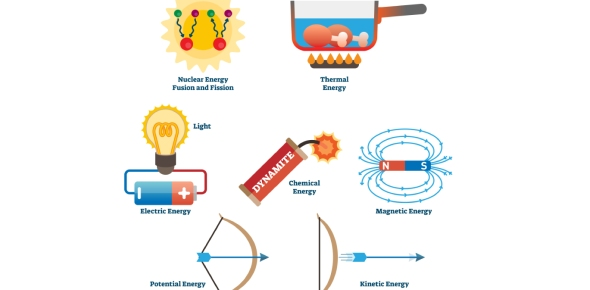# Chapter B3 Forms Of Energy

30 Questions | Attempts: 261
ShareSettings• 1.
The batteries in your iPod are an example of
• A.

Potential energy

• B.

Mechanical energy

• C.

• D.

Nuclear energy

• 2.
An electric drill changes electrical energy into
• A.

Chemical energy

• B.

Mechanical energy

• C.

Thermal energy

• 3.
The ability to do work is
• 4.
What is the only type of radiant energy you can see?
• A.

X-ray

• B.

Gamma rays

• C.

Visible light

• D.

• 5.
A wound spring is an example of
• A.

Potential energy

• B.

Kinetic energy

• 6.
What separates white light into different wavelengths?
• A.

Magnifying glass

• B.

Prism

• C.

Flashlight

• D.

Microscope

• 7.
Bats make low frequency sounds.
• A.

True

• B.

False

• 8.
What type of energy can cause a sunburn?
• A.

Gamma rays

• B.

Ultraviolet light

• C.

Microwaves

• D.

X-rays

• 9.
If you drop a ball from the top of a building, where does it have the most potential energy?
• A.

When it hits the ground

• B.

Halfway down

• C.

At the top of the building

• 10.
When you turn on the radio you change electrical energy into
• A.

Mechanical energy

• B.

Sound energy

• C.

Electrical energy

• D.

Nuclear energy

• 11.
What characteristic do we use to classify different types of radiant energy?
• 12.
A skier has the most kinetic energy
• A.

At the bottom of the slope

• B.

At the top of the slope

• C.

Halfway down the slope

• 13.
Sound energy needs matter to
• 14.
We say a material is translucent when
• A.

All the light that shines on it passes through and you can see clearly through it

• B.

Some of the light that shines on it passes through and you can see though it but not clearly

• C.

No light can pass through the material

• 15.
What type of energy travels in waves through empty space?
• A.

Sound

• B.

Mechanical

• C.

• 16.
What type of material allows no visible light to pass through it?
• A.

Transparent

• B.

Translucent

• C.

Opaque

• 17.
What type of radiant energy would you use to find out if you broke your leg skiing?
• A.

Gamma rays

• B.

X-rays

• C.

Microwaves

• D.

• 18.
What kind of material allows visible light to travel though it easily allowing a person to see clearly?
• A.

Transparent

• B.

Opaque

• C.

Translucent

• 19.
The energy of motion is
• A.

Kinetic energy

• B.

Potential energy

• 20.
The number of waves passing a point in a period of time is its
• 21.
The rapid back and forth movement of matter necessary for sound energy to travel is called
• 22.
Energy due to the movement of atoms or molecules is
• 23.
The energy an object has due to its motion, position or condition is
• A.

Mechanical energy

• B.

Electrical energy

• C.

Chemical energy

• 24.
The food we eat is an example of
• A.

Mechanical energy

• B.

Electrical energy

• C.

Chemical energy

• D.

• 25.
Humans can hear all frequencies of sound.
• A.

True

• B.

False

## Related TopicsBack to top
×

Wait!
Here's an interesting quiz for you.